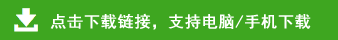# 小学四年级上册数学计算能力过关测试卷

一、直接写出得数。（16分，每题1分）

240÷40 =400÷80 =13×6 =        96÷4 =

39＋46=         40×50=65－27=90÷5=

360＋90=        97-79=          96－32÷16=      137-75-25

56÷7×8=       2×5÷2×5=     72÷(12×3)=     48－18÷6=

1.根据85×32=2720，直接写出下面各题的积。（4分）

85×64=         85×16=          85×8=       85×320=

780÷60=        2400÷200=       540÷30=       650÷50=

2.估算下面各题（4分）

52×98≈        69×103≈        161×28≈      32×103≈

3.已知A×B=380，如果A扩大3倍，,B不变，则积是（    ）；